Multiple choice

What is the flag pattern?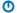A

Use a variable to detect if one or more of several things happened.

B

Marks the country of origin of a data file.

C

A visual indicator that a large file transfer is complete.

D

What Cthulhu waves to start a goat race.

Not graded. So why do it?

Lesson contents

### Error handling could be better

Right now, if there's an error, we show a `MsgBox`, and stop the program. Here's an example of a gender check:

• tGender = Cells(3, 4)
• tGender = Trim(LCase(tGender))
• If tGender <> "f" and tGender <> "m" Then
•     MsgBox "Sorry, gender must be F or M."
•     End
• End If

It works, but it isn't great. Usually, people are typing in more than one input, like this from the last lesson: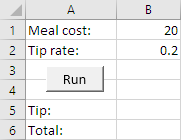Suppose a user types incorrect values in both fields. The program tells them about the first error, and stops. They fix it, and run the program again. The program fails again, telling them about the second error. They might think to themself:

Self, why didn't the stupid program check both inputs at once, and tell me all of the errors? Then I could have fixed everything at the same time.

So that's one thing.

Another problem is that the error messages are ephemeral, that is, they vanish quickly. Suppose you ran the tip program, and got this error: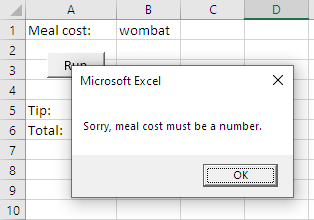You click OK, the message vanishes, and you get ready to fix the error. Before you do, your phone dings, with a new Discord message. You read it, reply, and turn back to your PC. By this time, you're forgotten what the problem was, and there's nothing on the screen to remind you. Drat!

A third problem has to do with output staying around for too long. You run the tip program once, with good data, and get a good result.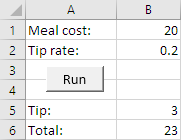You copy the output into an email, or whatever you want to do with it.

You want to do another calculation, so you put in new data.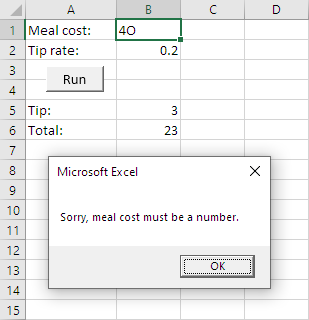You accidentally type `4O` - that's an uppercase O - instead of `40`. You get an error message, and click OK, so your screen looks like: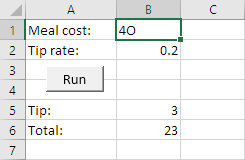The output from last time is still there.

Before you fix the error, your drunk boss wanders in, says a few stupid bossy things, and wanders out. You turn back to your PC, and see the screen above. You've forgotten about the error. You email the output to someone. Problem is: it's the wrong output!

Your drunk boss fires you. You end up homeless, and accidentally wander into Cthulhu's goat-filled paradise. Cthulhu won't be pleased to see you there.### Clearing output

Let's fix it. We'll start with the last problem. Let's make sure that if there are any errors, no output shows. So, here was our first run:All OK.

You type `4O` into the meal cost, and click Run. You get: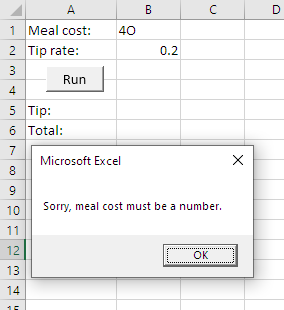Check out cells B5 and B6. No output! Nothing to deceive you. Yay!

This is particularly easy to do. Just add two lines of code at the start of the program.

• ...
• Dim sTotal As Single
•
• 'Clear previous output
• Cells(5, 2) = ""
• Cells(6, 2) = ""
•
• 'Validate meal cost
• tUncheckedUserInput = Cells(1, 2)
• ...

That's it! When the program runs, it clears the output cells. If there are errors, the cells will stay empty.Georgina

That's so cool! Simple.

So that's one problem: removing bad output. Now let's make the error messages last.

### Error messages that hang around

Instead of showing error messages in dialogs, let's show them on the worksheet. Like this: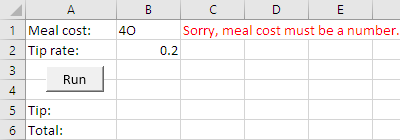Boss interrupts, whatevs, the message is still there. M0000t!

Here's our code so far:

• 'Validate meal cost
• tUncheckedUserInput = Cells(1, 2)
• If Not IsNumeric(tUncheckedUserInput) Then
•     MsgBox "Sorry, meal cost must be a number."
•     End
• End If

Change how the error is reported. Like this:

• 'Validate meal cost
• tUncheckedUserInput = Cells(1, 2)
• If Not IsNumeric(tUncheckedUserInput) Then
•     Cells(1, 3) = "Sorry, meal cost must be a number."
•     Cells(1, 3).Font.Color = vbRed
•     End
• End If

Not right yet, though. Fix the `4O` input error, run the program again, and you get: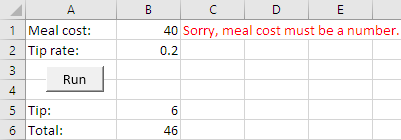The error message from last time is still there!

No problem. We already have code that clears the output when the program starts. Clear the error messages as well.

• ...
• Dim sTotal As Single
•
• 'Clear previous output
• Cells(5, 2) = ""
• Cells(6, 2) = ""
• 'Clear error messages
• Cells(1, 3) = ""
• Cells(2, 3) = ""
•
• 'Validate meal cost
• tUncheckedUserInput = Cells(1, 2)
• ...

So far, we know how to:

• Show an error message in a cell of the worksheet.
• Clear the error message when the program starts.

Yay! Better!

More to do, though.

### Show all errors at once

We've dealt with two issues do far:

• Clearing old output
• Making error messages hang around

The last issue is to show all of the error messages at once. We want something like this.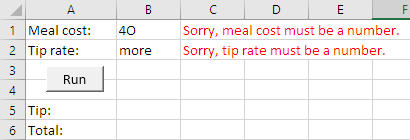The problem is that pesky `End`:

• 'Validate meal cost
• tUncheckedUserInput = Cells(1, 2)
• If Not IsNumeric(tUncheckedUserInput) Then
•     Cells(1, 3) = "Sorry, meal cost must be a number."
•     Cells(1, 3).Font.Color = vbRed
•     End
• End If

It ends the program as soon as the first error is detected. It's kind of right. We want to end the program, just not immediately. We want to run all of the error tests, then end the program if there has been an error with any inputs.

Use a flag. A flag is a variable showing the result of a bunch of code. In this case, the result of error checks.

You might remember Flag Goat from earlier.​Hello, again!

We'll use the flag pattern, to do all of the error checks, and track whether any of them failed.

Pattern

A variable that summarizes the results of a bunch of code.

• Dim tIsInputOk As String
• ...
• 'Clear previous output
• Cells(5, 2) = ""
• Cells(6, 2) = ""
• Cells(1, 3) = ""
• Cells(2, 3) = ""
•
• tIsInputOk = "yes" Flag starts off saying that the data is OK
•
• 'Validate meal cost
• tUncheckedUserInput = Cells(1, 2)
• If Not IsNumeric(tUncheckedUserInput) Then
•     'Not numeric, show error.
•     Cells(1, 3) = "Sorry, meal cost must be a number."
•     Cells(1, 3).Font.Color = vbRed
•     tIsInputOk = "no" Error, change the flag
• Else
•     'Is numeric.
•     sMealCost = tUncheckedUserInput
•     If sMealCost < 0 Then
•         Cells(1, 3) = "Sorry, meal cost cannot be negative."
•         Cells(1, 3).Font.Color = vbRed
•         tIsInputOk = "no" Error, change the flag
•     End If
• End If
•
• 'Validate tip rate
• tUncheckedUserInput = Cells(2, 2)
• If Not IsNumeric(tUncheckedUserInput) Then
•     'Not numeric, show error.
•     Cells(2, 3) = "Sorry, tip rate must be a number."
•     Cells(2, 3).Font.Color = vbRed
•     tIsInputOk = "no" Error, change the flag
• Else
•     'Is numeric.
•     sTipRate = tUncheckedUserInput
•     If sTpRate < 0 Then
•         Cells(2, 3) = "Sorry, tip rate cannot be negative."
•         Cells(2, 3).Font.Color = vbRed
•         tIsInputOk = "no" Error, change the flag
•     End If
• End If
•
• 'Did anything go wrong?
• If tIsInputOk = "no" Then Test flag after all validation
•     End
• End If

We start off assuming that all of the input is OK.

• tIsInputOk = "yes"

Then, if any error is detected, the flag is set to "no".

• ...
• If Not IsNumeric(tUncheckedUserInput) Then
•     ...
•     tIsInputOk = "no" Error - set flag to false
•     ...
• Else
•     ...
•     If sMealCost < 0 Then
•         ...
•         tIsInputOk = "no" Error - set flag to false
•     End If
• End If
• ...
• If Not IsNumeric(tUncheckedUserInput) Then
•     ...
•     tIsInputOk = "no" Error - set flag to false
• Else
•     ...
•     If sTipRate < 0 Then
•         ...
•         tIsInputOk = "no" Error - set flag to false
•     End If
• End If

The last step is to stop the program, if there were any errors. This check goes after all of the input checks are finished.

• 'Did anything go wrong?
• If tIsInputOk = "no" Then
•     End
• End If

### Put it all together

There you have it.

• Clear old output when the program starts
• Clear old error messages when the program starts
• Set a flag
• If there's an error, show a message on the worksheet, and change the flag
• Do as many checks as you want
• After the last check, test the flag, to see if the program should stop

This gives us a new pattern, made up of other chunks.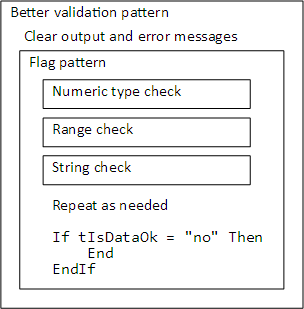Pattern

Wrap a bunch of individual checks inside the flag pattern.

### Exercises

Exercise

More dwarves?

You have some Snow Whites and some dwarves. Each Snow White needs seven dwarves, but you might have more or fewer dwarves than you need. Write a program to figure out how many dwarves to add, or get rid of.

It starts like this: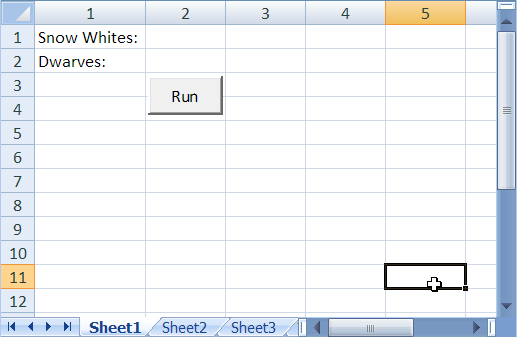Make sure that the two input values are numeric, and zero or more. Show errors in the worksheet: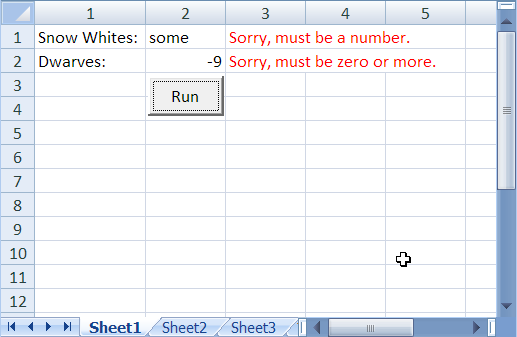Make sure that the errors and output from one run are cleared when the next run starts.

Here are output samples. Note that singular/plural is handled correctly. You'll needs some `If`s for this.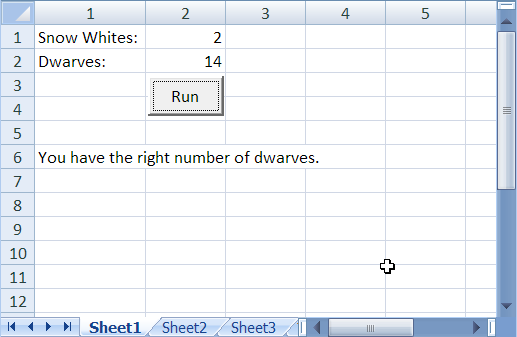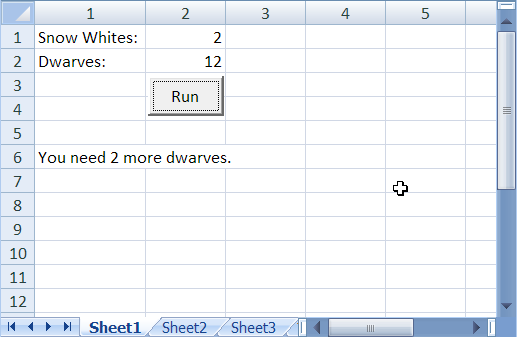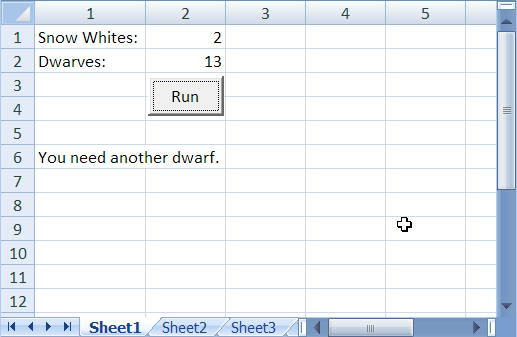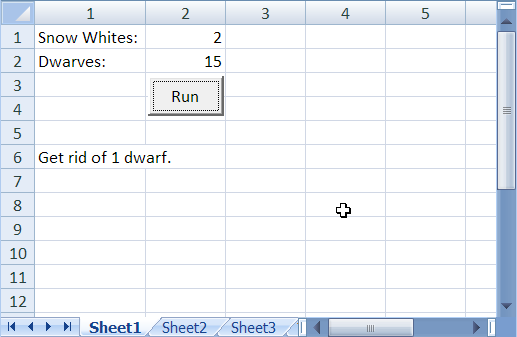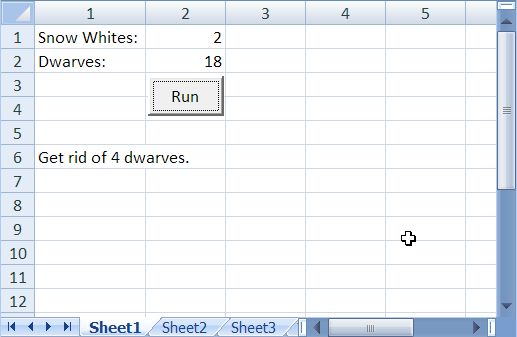``````dwarvesNeeded = snowWhites * 7